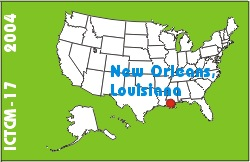# Animations using Maple

### Bill Blyth

Department of Mathematics and Statistics
RMIT University
GPO Box 2476V
Melbourne, 3001
Australia

list of all papers by this author

 Click to access this paper: paper.pdf

## ABSTRACT

We use the visualization and animation capabilities of Maple throughout our courses. Animations are used in presentations in class from first year onwards. For example, animations for Newton's method and for fixed point iteration; to illustrate how slicing is used to generate the domain for double integration; to show how the error function (for the collocation method solution of a boundary value problem) varies as the collocation points are changed.

This paper shows how to provide dynamically labeled animations. We start with a simple animation of parameterized curves where the value of the parameter is displayed on each frame. Our students in their first semester of first year asked to work individually or in pairs to attempt an animation assignment. The students are required to work in a group of size 3 plus or minus 1 and choose one of the 5 problems that are given. These problems are based on parameterized curves such as those that appear when modeling drug concentration in the blood. The presentation (in the lab) and assessment of each group's animation almost becomes a tutorial. This activity (which does not require calculus) is popular.

For our second example, we'll consider the standard calculus problem of finding the dimensions of the rectangle of maximum area that is inscribed in a triangle. Animation will be used to find the solution in a particular case. This solution can easily be shown to be the correct solution for the arbitrary case – no calculus is needed, only some simple algebra.

Finally, we briefly consider an advanced topic. From our third year Finite Element Methods FEM course, we briefly present an animation of the collocation method error function used to find very simply the 'optimal' collocation points (a very difficult task analytically).

Keyword(s): Maple, modeling, calculus